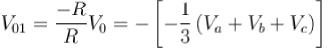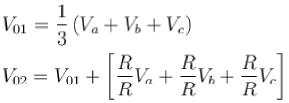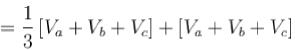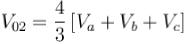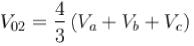Courses

# OP AMP MCQ Level – 2

## 10 Questions MCQ Test Topic wise Tests for IIT JAM Physics | OP AMP MCQ Level – 2

Description
This mock test of OP AMP MCQ Level – 2 for Physics helps you for every Physics entrance exam. This contains 10 Multiple Choice Questions for Physics OP AMP MCQ Level – 2 (mcq) to study with solutions a complete question bank. The solved questions answers in this OP AMP MCQ Level – 2 quiz give you a good mix of easy questions and tough questions. Physics students definitely take this OP AMP MCQ Level – 2 exercise for a better result in the exam. You can find other OP AMP MCQ Level – 2 extra questions, long questions & short questions for Physics on EduRev as well by searching above.
QUESTION: 1

### The circuit connection of Op-Amp given in the figure (i) and (ii) represent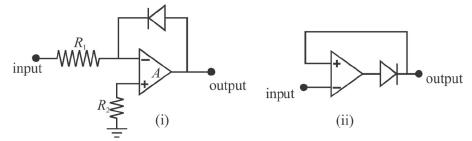Solution:

The circuit connection of Op-Amp given in figure (i) and (ii) represents logarithmic amplifier for figure (i) and detector for figure (ii)
The correct answer is: Logarithmic amplifier for figure (i) and detector for figure (ii)

QUESTION: 2

### Given the circuit below, calculate the output voltage of the non-inverting Op-Amp summer: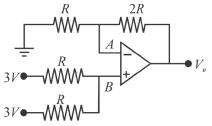Solution:

The output voltage of a non-inverting Op-Amp is.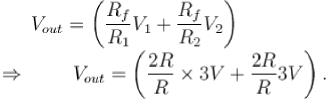= 12V

QUESTION: 3

### What should be the values of the component R and R2 such the frequency of the Wien bridge oscillator is 300Hz? (given C = 0.01 μf and R1 = 12kΩ.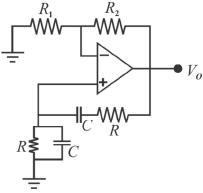Solution:

The frequency of oscillation f0 is exactly the resonant frequency of balanced Wien bridge and is given by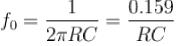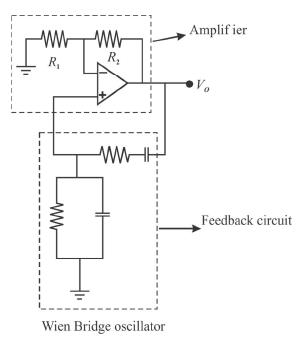Assuming that the resistor are equal in value, and the capacitors are equal is value in the reactive lag of the wien bridge. At the frequency, the gain required for sustained oscillation is given by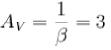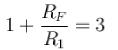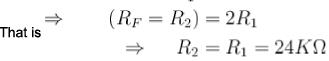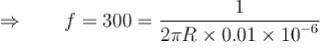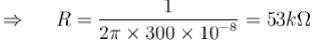The correct answer is: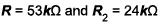QUESTION: 4

A non-inverting Op-Amp summer is shown in the figure. The output voltage V0 is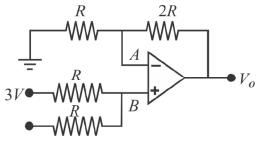Solution:

Potential at terminal B,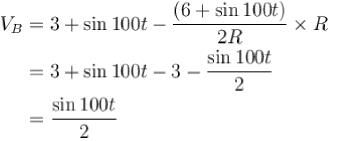Since, input impedence at Op-Amp is very large, the input current is negligible small and points A and B can be considered to be at same potential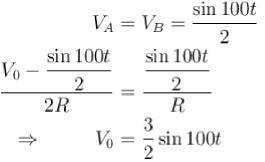The correct answer is: 3/2 sin 100t

QUESTION: 5

When negative feedback is used, then the gain bandwidth product of an Op-Amp.

Solution:

Applying the feedback will reduce the gain but increase the bandwidth hence, the gain bandwidth product = Ax f. he remains constant for any voltage feedback amplifier.
The correct answer is: stays the same

QUESTION: 6

The low-pass active filter shown in the figure has a cut-off frequency of 2kHz and a pass band gain of 1.5. The values of the resistors are :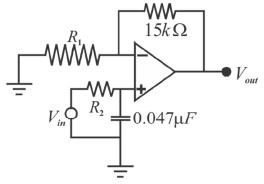Solution:

At f = fcc (cut o ff frequency at which gain falls to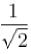of its low frequency value)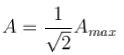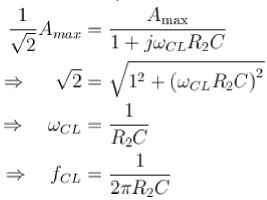(i) Pass-Band gain = Amax = 1.5.
Hence,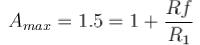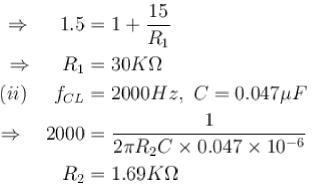The correct answer is: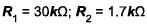QUESTION: 7

The circuit below represent a non-inverting integrator. Find the expression for the output voltage.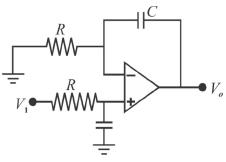Solution:

Let the inverting terminal of Op-Amp is at potential ‘V and hence non-inverting terminal also appears to have the same potential ‘V due to virtual ground concept.
Input current to op-amp is zero. Hence at non-inverting terminal mode, we have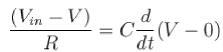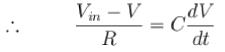Input current to Op-Amp is zero. Hence at inverting terminal mode we have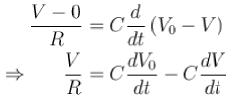Substituting the values and solving all the above equations, we get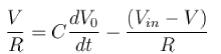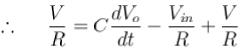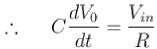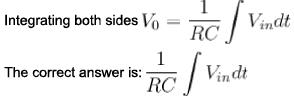QUESTION: 8

The transistor gain of the circuit shown in figure is given by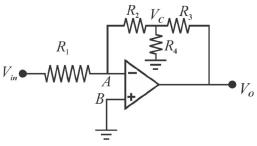Solution:

A and B can be measured at the same potential. From figure;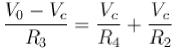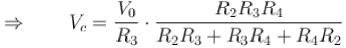or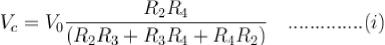Also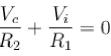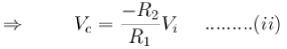Combing (i) and (ii)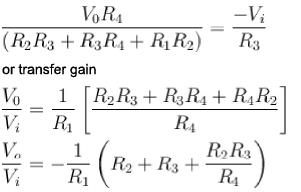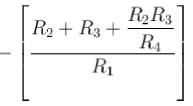QUESTION: 9

The output voltage at the end of second amplifier V01  is :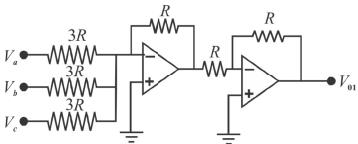The following circuit contains 3 Op-Amps and resistors.

Solution: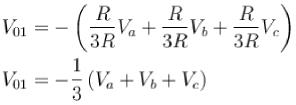Similarly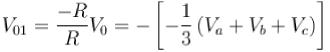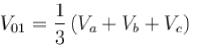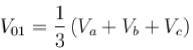QUESTION: 10

The output V02 (at the end of third Op-Amp) of the below circuit is :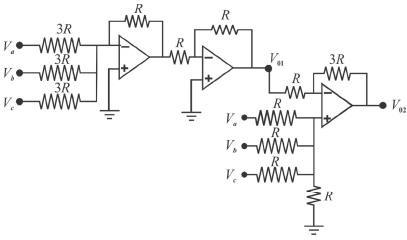Solution: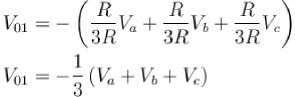Similarly